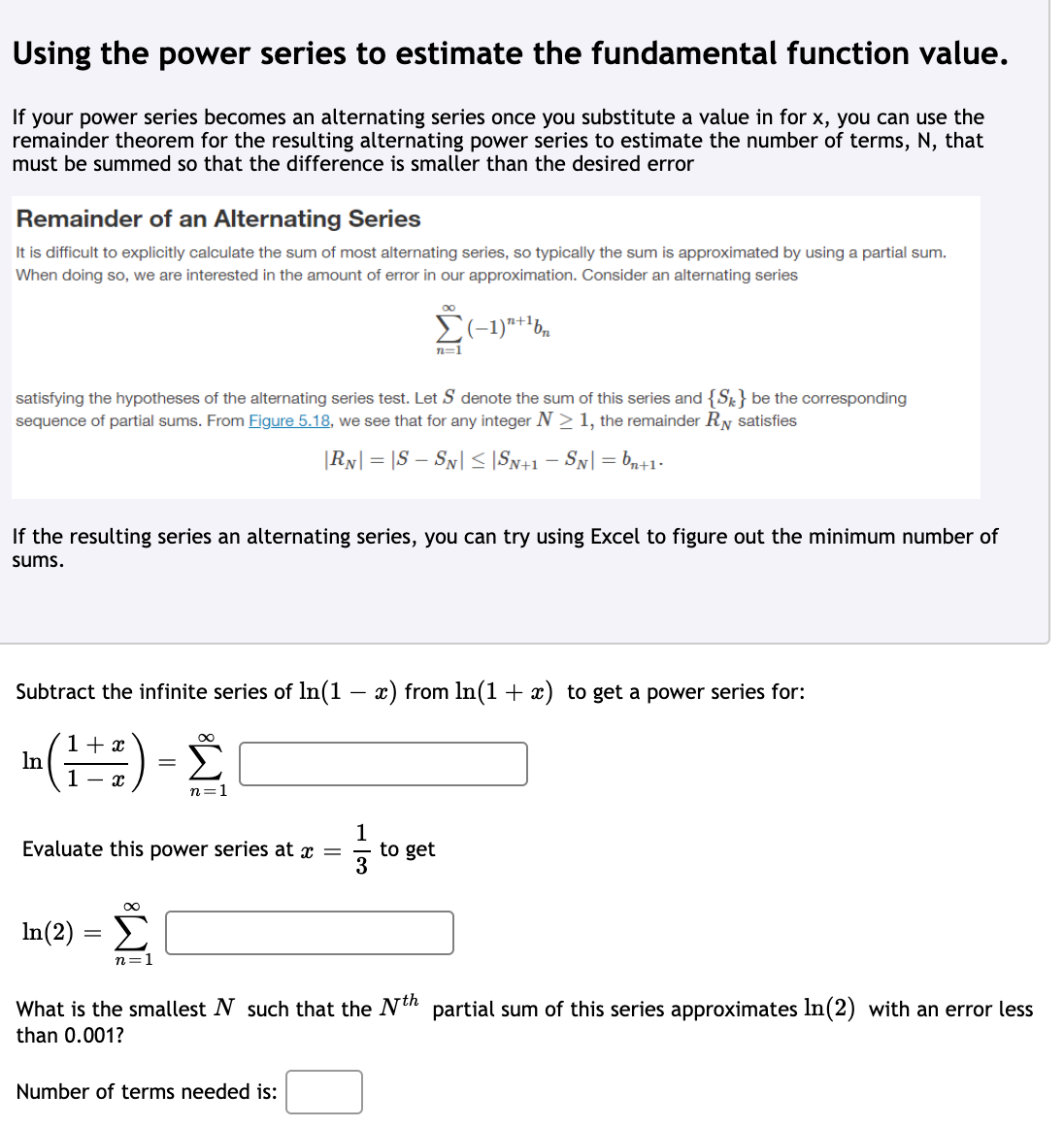# Subtract the infinite series of ln(1 – x) from In(1 + x) to get a power series for: + x Σ In %3D n=1 1 to get Evaluate this power series at x = In(2) = E n=1 What is the smallest N such that the Nth partial sum of this series approximates In(2) with an error less than 0.001? Number of terms needed is:

Questionhelp_outlineImage TranscriptioncloseSubtract the infinite series of ln(1 – x) from In(1 + x) to get a power series for: + x Σ In %3D n=1 1 to get Evaluate this power series at x = In(2) = E n=1 What is the smallest N such that the Nth partial sum of this series approximates In(2) with an error less than 0.001? Number of terms needed is: fullscreen

### Want to see this answer and more?

Experts are waiting 24/7 to provide step-by-step solutions in as fast as 30 minutes!*

*Response times may vary by subject and question complexity. Median response time is 34 minutes for paid subscribers and may be longer for promotional offers.
Tagged in
MathCalculus

### Functions